# 一类二次系统(II)类方程的奇点性态分析Singularity Analysis of a Class of Quadratic System (II) Equations

DOI: 10.12677/AAM.2018.77095, PDF, HTML, XML, 下载: 611  浏览: 1,952  科研立项经费支持

Abstract: In order to study the planar system, it is first required to find out the singular points, then analyze the singularity type and its stability. Therefore, the singularity behavior analysis plays an im-portant role in the planar quadratic system. In this paper, three different methods are used to an-alyze the singularities of a class of quadratic system (II) equations.

1. 引言

(I)类方程： $\stackrel{˙}{x}=-y+dx+l{x}^{2}+mxy+n{y}^{2},\text{\hspace{0.17em}}\stackrel{˙}{y}=x$

(II)类方程： $\stackrel{˙}{x}=-y+dx+l{x}^{2}+mxy+n{y}^{2},\text{\hspace{0.17em}}\stackrel{˙}{y}=x\left(1+ax\right),\text{\hspace{0.17em}}a\ne 0$

(III)类方程： $\stackrel{˙}{x}=-y+dx+l{x}^{2}+mxy+n{y}^{2},\text{\hspace{0.17em}}\stackrel{˙}{y}=x\left(1+ax+by\right),\text{\hspace{0.17em}}b\ne 0$

2. 预备知识

$\stackrel{˙}{x}=P\left(x,y\right),\text{\hspace{0.17em}}\stackrel{˙}{y}=Q\left(x,y\right)$ (2.1)

$F\left(\theta \right)={\int }_{0}^{\theta }f\left(s\right)\text{d}s=g\theta +\phi \left(\theta \right)$ (2.2)

1) F正定： $F\left(0,0\right)=0$$F\left(x,y\right)>0$ ，当 $\left(x,y\right)\ne \left(0,0\right)$

2) ${\frac{\text{d}F}{\text{d}t}|}_{\left(2.1\right)}<0\left(>0\right)$ ，当 $\left(x,y\right)\ne \left(0,0\right)$

${W}_{1}=m\left(l+n\right)-a\left(b+2l\right).$

${W}_{2}=ma\left(5a-m\right)\left[{\left(l+n\right)}^{2}\left(n+b\right)-{a}^{2}\left(b+2l+n\right)\right].$

${W}_{3}=m{a}^{2}\left[2{a}^{2}+n\left(l+2n\right)\right]\left[{\left(l+n\right)}^{2}\left(n+b\right)-{a}^{2}\left(b+2l+n\right)\right].$

1) 当 $d=0$${W}_{1}\ne 0$ ，则点 $O\left(0,0\right)$ 为一阶细焦点， ${W}_{1}<0$ 时点 $O\left(0,0\right)$ 为稳定， ${W}_{1}>0$ 时点 $O\left(0,0\right)$ 为不稳定；

2) 当 $d={W}_{1}=0$${W}_{2}\ne 0$ ，则点 $O\left(0,0\right)$ 为二阶细焦点， ${W}_{2}<0$ 时点 $O\left(0,0\right)$ 为稳定， ${W}_{2}>0$ 时点 $O\left(0,0\right)$ 为不稳定；

3) 当 $d={W}_{1}={W}_{2}=0$${W}_{3}\ne 0$ ，则点 $O\left(0,0\right)$ 为三阶细焦点， ${W}_{3}<0$ 时点 $O\left(0,0\right)$ 为稳定， ${W}_{3}>0$ 时点 $O\left(0,0\right)$ 为不稳定；

4) 当 $d={W}_{1}={W}_{2}={W}_{3}$ 时，点 $O\left(0,0\right)$ 为中心。

3. 主要结果

$d=l=0$$m=-a$$n=-1$ 时，(II)类方程化为 $\stackrel{˙}{x}=-y-axy-{y}^{2}$$\stackrel{˙}{y}=x+a{x}^{2}$

$O\left(0,0\right),\text{\hspace{0.17em}}A\left(0,-1\right),\text{\hspace{0.17em}}B\left(-\frac{1}{a},0\right),\text{\hspace{0.17em}}C\left(-\frac{1}{a},-2\right).$

1) 由于 $O\left(0,0\right)$ 是对应线性系统的中心，对其非线性系统在原点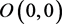的性态分析，本文给出三种不同的方法判断原系统在原点的性态，对于在点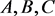处的奇点性态，要作一线性变换，把它先移到原点进而再判断其稳定性态。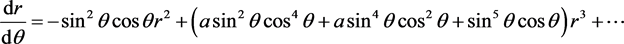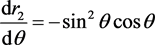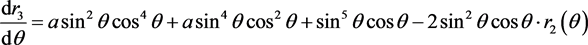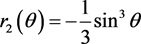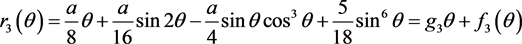，至此便可判定原点为原系统的一阶细焦点，当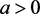时为不稳定，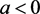时为稳定。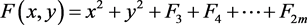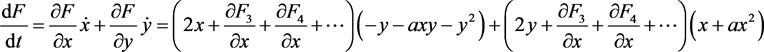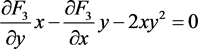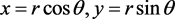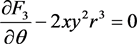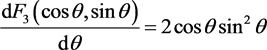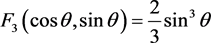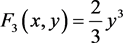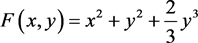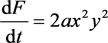2) 接下来我们对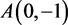进行分析，首先作一线性变换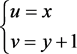，得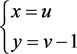是鞍点，此解是不稳定的。

3) 同理，对于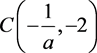，作一线性变换，令，得4) 对于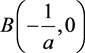，作一线性变换，令，得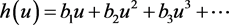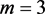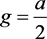。故而当时原点为不稳定结点；当时原点为鞍点。

  罗定军, 张详, 董梅芳. 动力系统的定性与分支理论[M]. 北京: 科学出版社, 2001.  张锦炎, 冯贝叶. 常微分方程几何理论与分支问题[M]. 北京: 北京大学出版社, 2000.  张芷芬,丁同仁, 黄文灶. 微分方程定性理论[M]. 北京: 科学出版社, 1985.  叶彦谦. 多项式微分系统定性理论[M]. 上海: 上海科学技术出版社, 1995.  韩茂安, 朱德明. 微分方程分支理论[M]. 北京: 煤炭工业出版社, 1994.  王高雄, 周之铭, 王寿松. 常微分方程[M]. 北京: 高等教育出版社, 2006.